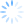We offer two Excel templates, using our software CDXZipStream or web service CDXGeoData, for calculating driving distance or time for a matrix (array) of locations.  A typical application for these templates would be calculating route data for a matrix of customers and stores.  Here is a short tutorial showing how this works with our web service CDXGeoData:

The template shown above automatically calculates driving distance or driving time for all combinations of locations listed. Once the calculations are complete, we can now go one step further and use simple Excel formulas to identify the specific store closest to each customer. In this case the store locations in the tutorial above are listed in row 9, in columns C through I, and customer addresses are listed in column B, starting in row 10.

To the right of the matrix data, enter the following in cell J10 for the first customer address:

=MATCH(MIN(C10:I10),C10:I10,0)+2

This equation identifies the column location of the lowest numerical value in cells C10 through I10, i.e. the shortest driving time for the first customer.  Note that the value “2” is added here since the data starts in the third column C.

To the right of the above equation, in cell K10, enter:

This equation identifies the store address (in row 9) associated with the shortest driving time identified by the previous equation in cell J10.

Now just copy these equations along the rest of the customer list, and the address of the closest store is identified for each customer.

Note: If desired, the equations above can be combined like this into one cell: